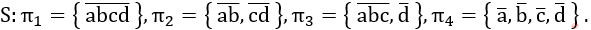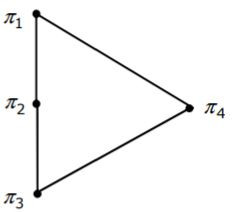## Sets-And Relation

 Question 1

Let R be the set of all binary relations on the set {1,2,3}. Suppose a relation is chosen from R at random. The probability that the chosen relation is reflexive (round off to 3 decimal places) is _____.

 A 0.125
Engineering-Mathematics       Sets-And Relation       GATE 2020       Video-Explanation
Question 1 Explanation:
For a set with n elements,
The number of reflexive relations is 2^(n^2-n).
The total number of relations on a set with n elements is 2^ (n^2).
The probability of choosing the reflexive relation out of set of relations is
= 2^(n^2-n) /2^ (n^2)
= 2^( n^2-n- n^2)
= 2^(-n)
Given n=3, the probability will be 2-n = ⅛ = 0.125
 Question 2

Let (S, ≤) be a partial order with two minimal elements a and b, and a maximum element c. Let P: S → {True, False} be a predicate defined on S. Suppose that P(a) = True, P(b) = False and P(x) ⇒ P(y) for all x, y ∈ S satisfying x ≤ y, where ⇒ stands for logical implication. Which of the following statements CANNOT be true?

 A P(x) = True for all x ∈ S such that x ≠ b B P(x) = False for all x ∈ S such that x ≠ a and x ≠ c C P(x) = False for all x ∈ S such that b ≤ x and x ≠ c D P(x) = False for all x ∈ S such that a ≤ x and b ≤ x
Engineering-Mathematics       Sets-And Relation       GATE 2003
Question 2 Explanation:
c is the maximum element.
a or b the minimal element in set.
P(a) = True for all x ∈ S such that a ≤ x and b ≤ x.
Option D is False.
 Question 3

Let A, B and C be non-empty sets and let X = (A - B) - C and Y = (A - C) - (B - C). Which one of the following is TRUE?

 A X = Y B X ⊂ Y C Y ⊂ X D None of these
Engineering-Mathematics       Sets-And Relation       GATE 2005
Question 3 Explanation:
Consider, A = {1, 2, 3, 4, 5, 6}
B = {1, 3, 4, 5}
C = {2, 4, 5, 6}
X = (A - B) - C
X = {2, 6} - {2, 4, 5, 6}
= ∅
Y = (A - C) - (B - C)
= {1, 3} - { 1, 3}
= ∅
X = YX = (A - B) - C
= (1, 5) - (5, 7, 4, 3)
= (1)
Y = (A - C) - (B - C)
= (1, 4) - (2, 4)
= (1)
X = Y
 Question 4

The set {1, 2, 4, 7, 8, 11, 13, 14} is a group under multiplication modulo 15. The inverses of 4 and 7 are respectively:

 A 3 and 13 B 2 and 11 C 4 and 13 D 8 and 14
Engineering-Mathematics       Sets-And Relation       GATE 2005
Question 4 Explanation:
Let say,
Inverse of 4 = m; Inverse of 7 = n
(4×m)%15=1; (7*n)%15=1
Option A: m=3 n=13
12%15≠1 (✖️) 91%15=1 (✔️)
Option B: m=2 n=11
8%15≠1 (✖️) 11%15≠1 (✖️)
Option C: m=4 n=13
16%15=1(✔️) 91%15=1 (✔️)
Option D: m=8 n=14
120%15≠1(✖️) 98%15≠1(✖️)
 Question 5

The set {1, 2, 3, 5, 7, 8, 9} under multiplication modulo 10 is not a group. Given below are four plausible reasons. Which one of them is false?

 A It is not closed B 2 does not have an inverse C 3 does not have an inverse D 8 does not have an inverse
Engineering-Mathematics       Sets-And Relation       GATE 2006
Question 5 Explanation:
The given set is x = {1,2,3,5,7,8,9}
Option A:
It is not closed under multiplication. After multiplication modulo (10) we get ‘0’. The ‘0’ is not present in the set.
(2*5)%10 ⇒ 10%10 = 0
Option B:
2 does not have an inverse such as
(2*x)%10 ≠ 1
Option C:
3 have an inverse such that
(3*7)%10 = 1
Option D:
8 does not have an inverse such that
(8*x)%10 ≠ 1
 Question 6

Consider the set S = {a,b,c,d}. Consider the following 4 partitions π1, π2, π3, π4 onLet p be the partial order on the set of partitions S' = {π1, π2, π3, π4} defined as follows: πi p πj if and only if πi refines πj. The poset diagram for (S', p) is:

 ABCDEngineering-Mathematics       Sets-And Relation       GATE 2007
Question 6 Explanation:
π4 = refines every partition. So it has to be bottom of poset diagram.
And, neither π2 refines π3, nor π3 refines π2.
Here, only π1 refined by every set, so it has to be at the top.
Finally, option C satisfies all the property.
There are 6 questions to complete.

Register Now